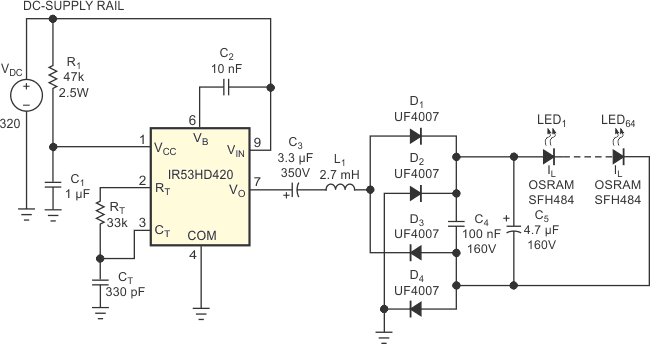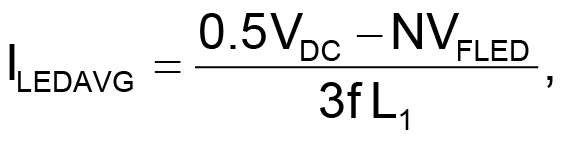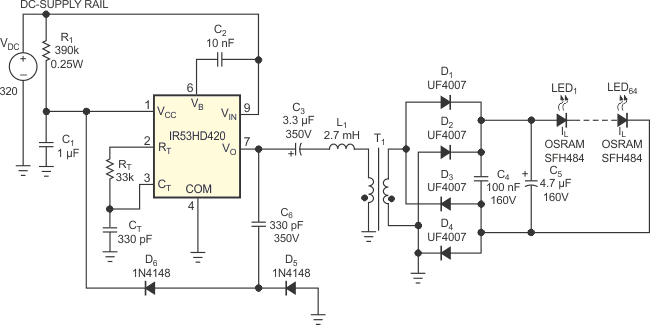# Use a CFL ballast to drive LEDs

## International Rectifier IR53HD420

Christian Rausch

EDN

Designers use ballast ICs, such as International Rectifier’s IR53HD420, in CFLs (compact fluorescent lamps) for heating the filaments, igniting the lamps, and supplying the lamps with current. Manufacturers produce these ICs in high volumes, and they cost approximately \$2. This Design Idea shows how you can use a CFL-ballast IC for driving LEDs instead of CFLs. A ballast IC essentially is a self-oscillating half-bridge for offline operation. It typically operates from 320 V dc, which is approximately the same power as that from a 230 V-ac mains rectifier or a 120 V voltage doubler. The IC generates square-wave voltages with an amplitude of 320 V p-p and a frequency of tens of kilohertz.

Usually, this square-wave voltage connects to a series combination of a CFL tube and a current-limiting inductor, L1 (Figure 1). Together with a parallel capacitor and using the LC resonance, you can warm up, ignite, and supply the tube with current. This approach works well because CFL tubes have high impedances when they are off and low impedance when they are running. The tube voltage is typically 150 V p-p.Figure 1. A CFL ballast drives a long string of LEDs.

By putting several LEDs in series and connecting them to a bridge rectifier, you can effect an imitation of a CFL, at least in the on-state. Imitating the off-state is less important, because LEDs need no ignition procedure. At the given values for RT and CT, the bridge runs at 70 kHz. The circuit supplies 64 LEDs with a current of approximately 80 mA. The infrared LEDs illuminate the field of view of a CCD camera in a machine-vision system. The circuit prototype uses a 2.7-mH inductor from a dead CFL.

The LED current comprises dc current plus a small ripple current; keep the ripple current low for high efficiency and long LED lifetime. LED manufacturers usually demand values of a few percentage points. Such a low ripple current may be difficult to achieve with one electrolytic capacitor, C5, but a parallel combination with an additional foil capacitor, C4, works well enough in most cases. The voltage at the input of the LED rectifier is fairly constant during one oscillation period, so the inductor current has a triangular shape, which is good for EMC (electromagnetic compatibility). The equation for the average LED current iswhere VDC is the supply voltage, N is the number of LEDs in series, VFLED is the LED forward voltage, f is the oscillation frequency, and L1 is the inductance of the current-limiting inductor.

Although the circuit of Figure 1 works well, it has some deficiencies that the circuit of Figure 2 remedies by adding C6, D5, D6, and T1, wound on an EPCOS EP13 coil former, with an ungapped-EP13-core of T38 material with an inductance of 7000 nH. Both the primary and the secondary windings are 90 turns of 0.2-mm wire; the secondary winding is wound on top of the primary winding. Stray inductance is not important in this case, and the inductance for both the primary and the secondary windings is 50 mH. The circuit in Figure 2 has several advantages over the one in Figure 1. For example, the supply current for the ballast IC of Figure 1 must flow through R1 and into the IR53HD420, where it gets clamped to 15.6 V. At a supply current of about 6 mA, R1 must dissipate more than 2 W. In Figure 2, R1 can have a much higher value, because it must supply only a small start-up current. After start-up, a charge pump comprising C6, D5, and D6 pumps enough current into the VCC pin so that the internal zener diode clamps to 15.6 V. The design equation for the charge pumps average current isThe dissipation of R1 now stays below 0.25 W.Figure 2. Adding a transformer to the circuit in Figure 1 allows you to connect as many LEDs as necessary.

Also, the summed forward voltages of the LEDs in Figure 1 must be smaller than one-half the supply voltage. For the circuit in Figure 2, by tailoring the transformer-winding ratio, you can connect as many LEDs as needed, as long as you do not exceed the ratings of the components. (LED voltages even higher than VDC are possible.) A less obvious problem of the circuit in Figure 1 is that the full voltage swing of the bridge appears at both ends of the LED string. This situation does not present a problem when all the LEDs are close together and the LEDs are close to the bridge. However, in many light fixtures, you wish to separate the LEDs from the electronics. Due to stray capacitances, this approach would lead to high capacitive currents from the LEDs to ground, corrupting the efficiency and producing EMC problems. With the transformer of Figure 2, you can ground one end of the LED string either directly, as shown, or through a capacitor. Now, you can use long cables to easily separate the LEDs from the electronics.

EDN